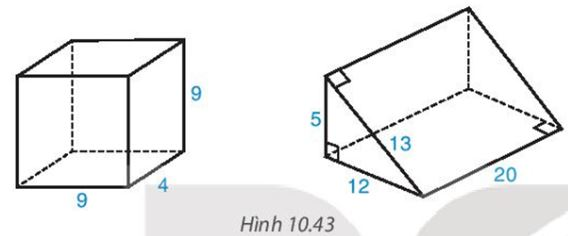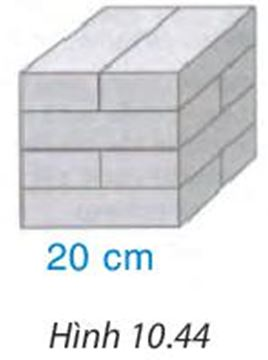## Solve the exercises at the end of Chapter X (Chapter 10 Math 7 Connect) – Math Book

Solve the exercises at the end of Chapter X (Chapter 10 Math 7 Connect)

### Solution 10.20 page 102 Math 7 Textbook Connecting knowledge volume 2 – KNTT

A box is made in the form of a rectangular box out of cardboard with a length of 20 cm, a width of 14 cm and a height of 15 cm.

a) The volume of the box.

b) Calculate the area of ​​the cover used to make the box.

Detailed instructions for solving problems 10.20

Solution method

Area of ​​rectangle $${S_{xq}} = 2\left( {a + b} \right).c$$

Surrounding area of ​​cube: $${S_{xq}} = 4{a^2}$$.

Volume of rectangular box $$V = abc$$.

Volume of cube $$V = {a^3}$$.

Detailed explanation

a) The volume of the box is:

20. 14. 15 = 4200 (cm3)

b) The area of ​​the cover to make the box corresponds to the surrounding area and the area of ​​​​the 2 bottom faces of the rectangular box

The area of ​​the cover used to make the box is:

2. ( 14 + 20 ). 15 + 2. 20. 14 = 1580 (cm2)

–>

— *****

### Solve problem 10.21 page 102 Math 7 textbook Connecting knowledge volume 2 – KNTT

Calculate the volume, perimeter, and total area of ​​the rectangular box and prism in Figure 10.43Detailed instructions for solving problems 10.21

Solution method

– Area of ​​rectangular box $${S_{xq}} = 2\left( {a + b} \right).c$$

– Volume of rectangular box $$V = abc$$.

– Surrounding area of ​​triangular vertical prism, quadrilateral vertical prism: $${S_{xq}} = Ch$$

– Volume of triangular vertical prism, tetragonal vertical prism: $$V = {S_{day}}.h$$

Detailed explanation

The area around the rectangular box is:

2. (4 + 9). 9 = 234

The total area of ​​the rectangular box is:

234 + 2 . 9 . 4 = 306

The volume of the rectangular box is:

9 . 4 . 9 = 324

The area around the prism is:

20 . ( 5 + 12 + 13 ) = 600

The total area of ​​the prism is:

600 + 2 . $$\frac{1}{2}$$ . 5 . 12 = 660

The volume of the rectangular box is:

20 x $$\frac{1}{2}$$ x 5 x12 = 600

–>

— *****

### Solve problem 10.22 page 102 Math 7 textbook Connecting knowledge volume 2 – KNTT

A number of bricks are arranged in the shape of a rectangular box to form a cube of side 20 cm as shown in Figure 10.44.

a) Calculate the perimeter and total area of ​​the cube.

b) Find the size of each brick.Detailed instructions for solving Lesson 10.22

Solution method

– Surrounding area of ​​cube: $${S_{xq}} = 4{a^2}$$.

– Volume of cube $$V = {a^3}$$.

– Area of ​​rectangular box $${S_{xq}} = 2\left( {a + b} \right).c$$

– Volume of rectangular box $$V = abc$$.

– Surrounding area of ​​triangular vertical prism, quadrilateral vertical prism: $${S_{xq}} = Ch$$

– Volume of triangular vertical prism, tetragonal vertical prism: $$V = {S_{day}}.h$$

Detailed explanation

a) The area around the cube block is:

4 . 202 = 1600 (cm2)

The area of ​​the bottom surface of the cube is:

20 . 20 = 400 (cm2)

The total area of ​​the cube is:

1600 + 2 . 400 = 2400 (cm2)

b) According to the figure, the width of the rectangular brick is equal to $$\frac{1}{2}$$ next to the cube

The width of the rectangular box is:

20 : 2=10 (cm)

The height of the brick is equal to $$\frac{1}{4}$$ side of the cube

The height of the brick is:

20:4=5 (cm)

So each brick has dimensions: length 20cm, width 10cm, height 5cm.

–>

— *****

### Solution 10.23 page 102 Math 7 Textbook Connecting knowledge volume 2 – KNTT

A rectangular room has a length of 5 m, a width of 4 m and a height of 3 m. People want to roll paint the walls and ceiling. Ask the area to be painted in square meters, knowing that the total area of ​​​​the doors is 5.8 m2 ?

Detailed instructions for solving Lesson 10.23

Solution method

– Area of ​​rectangular box $${S_{xq}} = 2\left( {a + b} \right).c$$

– Surrounding area of ​​triangular vertical prism, quadrilateral vertical prism: $${S_{xq}} = Ch$$

Detailed explanation

The area around the room is:

2 . ( 5 + 4 ). 3 = 21 (m2)

The total area of ​​the room is:

21 + 2 . 5 . 4 = 61 (m2)

The area to be painted is the total area of ​​the room minus the area of ​​the doors, so the paint roller area is:

61 – 5.8 = 55.2 (m2)

–>

— *****

### Solve problem 10.24 page 102 Math 7 Textbook Connecting knowledge volume 2 – KNTT

A rectangular fish tank made of glass (without lid) is 80cm long, 50cm wide, 45cm high. The initial water level in the tank is 35 cm high.

a) Calculate the area of ​​glass used to make that fish tank

b) A decorative stone is placed in the tank completely submerged in water, the water level of the tank rises to 37.5 cm. Calculate the volume of the rock.

Detailed instructions for solving Lesson 10.24

Solution method

Area of ​​rectangle $${S_{xq}} = 2\left( {a + b} \right).c$$

Surrounding area of ​​cube: $${S_{xq}} = 4{a^2}$$.

Volume of rectangular box $$V = abc$$.

Volume of cube $$V = {a^3}$$.

Detailed explanation

a) The area around the fish tank is:

2 . (80 + 50) . 45 = 11700 (cm2)

The glass area needed to make the fish tank is the surrounding area and the area of ​​one bottom surface of the rectangular box, so the required glass area is:

11700 + ( 80 . 50 ) = 15700 (cm2)

b)

The additional height of the water level is :

37.5 – 35 = 2.5 (cm)

The volume of water that rises after the stone is thrown will be equal to the volume of the stone, so the volume of the stone is:

4000 x 2.5 = 10000 (cm3 )

–>

— *****

### Solution 10.25 page 102 Math textbook 7 Connecting knowledge volume 2 – KNTT

A cylindrical cup filled with water. If you put 5 cubes of ice cubes of side 2 cm into the cup, how much water will come out?

Detailed instructions for solving Lesson 10.25

Solution method

Volume of cube $$V = {a^3}$$.

Detailed explanation

The volume of a stone is:

23 = 8 (cm3 )

The total volume of the 5 stones is:

8 . 5 = 40 (cm3 )

The volume of 5 ice cubes will be equal to the volume of water that rises after adding ice

=> The amount of water spilled will be 80 cm3 water.

–>

— *****